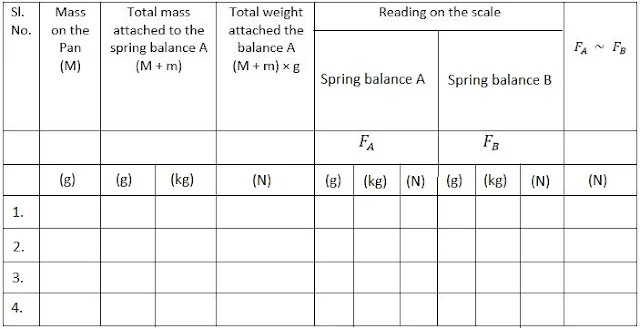#### To establish relationship between weight of a rectangular wooden block lying on a horizontal table and the minimum force required to just move it using a spring balance

Aim
To establish relationship between weight of a rectangular wooden block lying on a horizontal table and the minimum force required to just move it using a spring balance.

Theory
1. According to third law of motion, for every action, there is an equal and opposite reaction.
2. When one object exerts a force (action) on another object, then the second object also exerts a force (reaction) on the first.
3. These two forces are always equal in magnitude but in opposite direction.
4. The forces always exist in pairs.
5. The two opposing forces are known as action and reaction forces.
6. The forces of action and reaction always act on two different bodies.

Materials Required
Two identical spring balance, Thread, A pan of known mass, Weight box and A Motionless pulley

ProcedureStep 1: The range and the least count of the two spring balances is found.
Step 2: Ensured that the two spring balances are identical.
Step 3: The spring balances are checked and the force is measured.
Step 4: The two spring balances are hold vertically and ensured that their pointers are at zero mark.
Step 5: Both spring balances A and B, a pulley and a pan of known mass are arranged with the help of thread.
Step 6: The balance B must be attached to a rigid support.
Step 7: Now, the action and reaction forces are identified. The force which spring balance A exerts on B is action (reading on the scale of spring balance B). The reading on the scale of balance A shows the reaction that spring balance B exerts on A.
Step 8: Some mass M is put on the hanger. The total mass attached to the thread is now (M + m). The readings of both the balances are observed.
Step 9: The same activity as above is repeated with atleast four more masses on the pan of known mass. Table for the observations is formed.

Observation and Calculations
(i) Range of the two spring balances = .... N or g
(ii) Least count of the spring balance = .... N or g
(iii) Acceleration due to gravity (g) at your place = .... ms-2
(iv) Mass of the pan (given) m = .... g = .... kg.
(v) Weight of the pan w = m (in kg) × g = .... NPrecautions
1. The spring balances, pointer should are at zero mark.
2. The reading of the two spring balances should be noted only when their position conic to rest.
3.Spring balances should be identical.
4. The thread used should be inextensible.

Go to List of Experiments